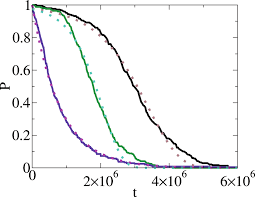## How to Calculate and Solve for P(A and B) | ProbabilityThe image above represents P(A and B).

To compute for P(A and B), four essential parameters are needed and these parameters are xA, NA, xB and NB.

The formula for calculating P(A and B):

P(A and B) = P(A) x P(B)

Where;

P(A) =  xANA
P(B) = xBNB

Let’s solve an example;
Find the P(A and B) when xA is 2, NA is 4, xB is 5 and NB is 8.

This implies that;

xA = 2
NA = 4
xB = 5
NB = 8

P(A and B) = P(A) x P(B)
P(A and B) = xANA x xBNB
P(A and B) = 24 x 58
P(A and B) = (2)(5)(4)(8)
P(A and B) = 1032
Divinding the numerator and denominator by 2
P(A and B) = 516
P(A and B) = 0.312

Therefore, the P(A and B) is 0.312.